### Indirect Standardization and Standardized Morbidity/Mortality Ratio

Indirect standardization compares the rates of the study and reference populations by applying the stratum-specific rates in the reference population to the study population, where the stratum-specific rates might not be reliable.

The expected number of events in the study population is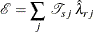where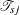is the population-time in the jth stratum of the study population and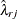is the rate in the jth stratum of the reference population.

With the expected number of events,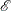, the standardized morbidity ratio or standardized mortality ratio can be expressed as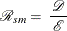where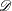is the observed number of events (Breslow and Day, 1987, p. 65).

The ratio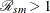indicates that the mortality rate or risk in the study population is larger than the estimate in the reference population, and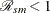indicates that the mortality rate or risk in the study population is smaller than the estimate in the reference population.

With the ratio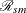, an indirectly standardized rate for the study population is computed as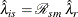where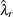is the overall crude rate in the reference population.

Similarly, to compare the risks of the study and reference populations, the stratum-specific risks in the reference population are used to compute the expected number of events in the study population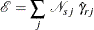where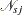is the number of observations in the jth stratum of the study population and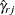is the risk in the jth stratum of the reference population.

Also, with the standardized morbidity ratio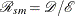, an indirectly standardized risk for the study population is computed as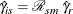where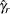is the overall crude risk in the reference population.

The observed number of events in the study population is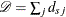, where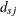is the number of events in the jth stratum of the population. For the rate estimate, ifhas a Poisson distribution, then the variance of the standardized mortality ratio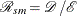is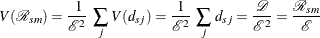For the risk estimate, ifhas a binomial distribution, then the variance ofis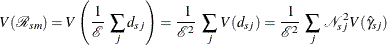where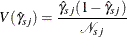By using the method of statistical differentials (Elandt-Johnson and Johnson, 1980, pp. 70–71), the variance of the logarithm ofcan be estimated by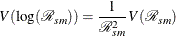For the rate estimate,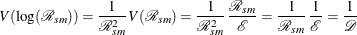The confidence intervals forcan be constructed based on normal, lognormal, and Poisson distributions.

#### Normal Distribution Confidence Interval for SMR

A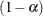confidence interval forbased on a normal distribution is given by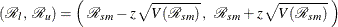where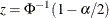is the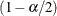quantile of the standard normal distribution.

A test statistic for the null hypothesis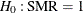is then given by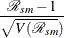The test statistic has an approximate standard normal distribution under.

#### Lognormal Distribution Confidence Interval for SMR

Aconfidence interval forbased on a normal distribution is given by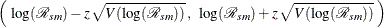whereis thequantile of the standard normal distribution.

Thus, aconfidence interval forbased on a lognormal distribution is given by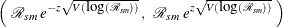A test statistic for the null hypothesisis then given by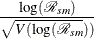The test statistic has an approximate standard normal distribution under.

#### Poisson Distribution Confidence Interval for Rate SMR

Denote the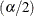quantile for thedistribution with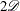degrees of freedom by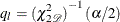Denote thequantiles for thedistribution with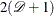degrees of freedom by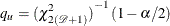Then aconfidence interval forbased on thedistribution is given by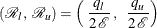A p-value for the test of the null hypothesisis given by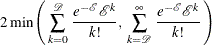#### Indirectly Standardized Rate and Its Confidence Interval

With a rate-standardized mortality ratio, an indirectly standardized rate for the study population is computed aswhereis the overall crude rate in the reference population.

Theconfidence intervals for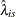can be constructed as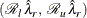where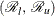is the confidence interval for.

#### Indirectly Standardized Risk and Its Confidence Interval

With a risk-standardized mortality ratio, an indirectly standardized risk for the study population is computed aswhereis the overall crude risk in the reference population.

Theconfidence intervals for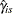can be constructed as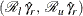whereis the confidence interval for.